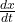Suppose a student carryinga virus returns toan isolated college campus o 1000 students. Determine a differential equation for the number x(t

Question

Suppose a student carryinga virus returns toan isolated college campus o 1000 students. Determine a differential equation for the number x(t) who have contracted the fluif the rate at which the disease spreads is proportional to the number of interactions between the number of students who have the fluand the number of students show have not been exposed to it.

in progress 0
5 months 2021-08-27T15:57:07+00:00 1 Answers 0 views 0= k.x.[1000 – x] ;

where k is the proportionality  constant

Step-by-step explanation:

Let

The number of people who are infected with the flu = x(t)

Total number of students = 1000

So,

Total number of student who do not expose with the flue = 1000 – x(t)

As given,

We have to find the differential equation such that

The rate at which the disease spreads is proportional to the number of interactions between the number of students who have the flu and the number of students show have not been exposed to it.∝ x(t) . [ 1000 – x(t) ]= k.x.[1000 – x] ; where k is the proportionality  constant